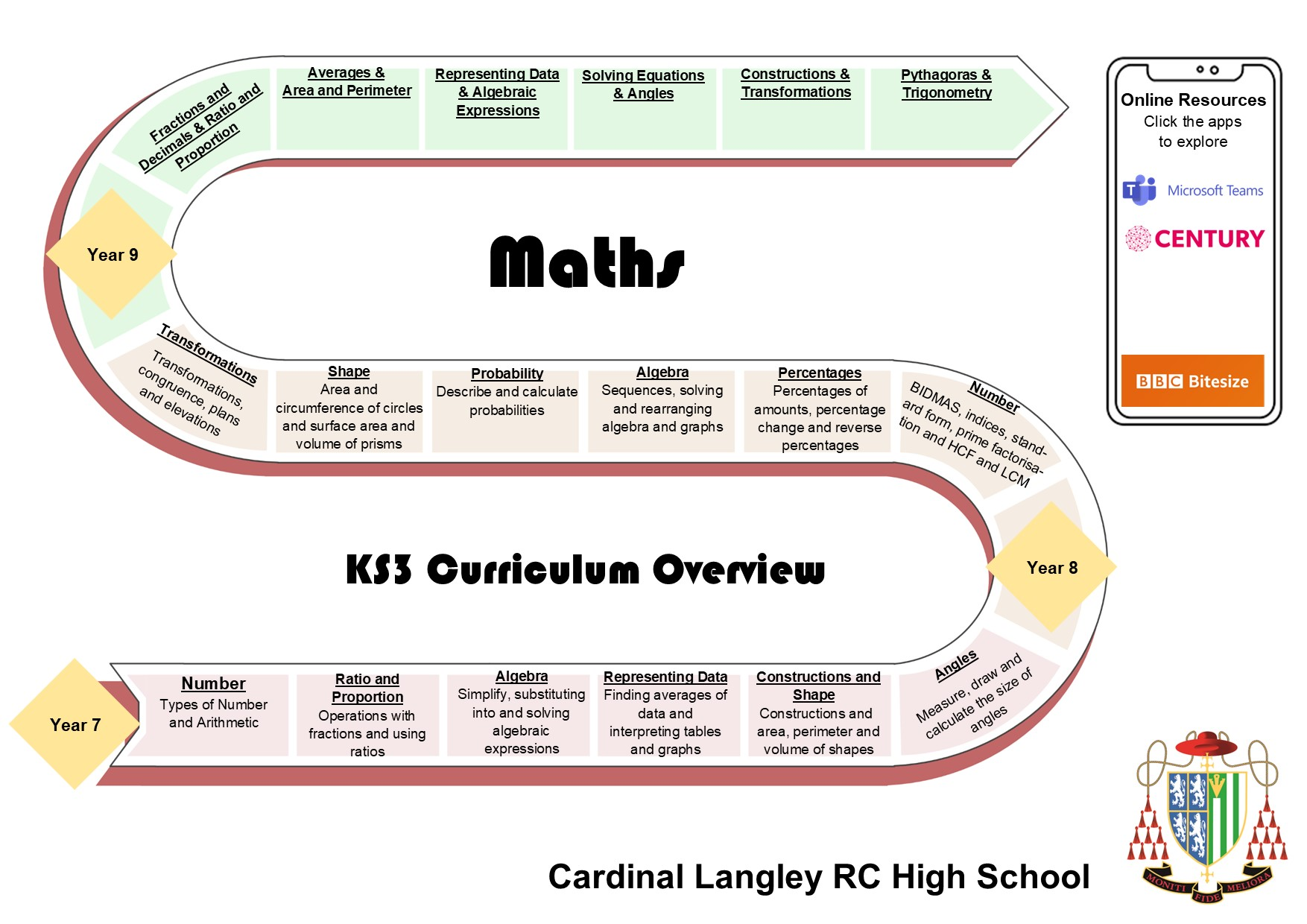## Mathematics

It was Galileo who said “Mathematics is the alphabet with which God created the universe”.

Mathematics is a core subject and encourages students to develop problem-solving skills and become effective and independent learners. With the focus on the functional elements of mathematics, applying mathematics in context, problem solving and reasoning, students learn to function mathematically in the world.

### Key Stage 3

In Key Stage 3, students are put into light streaming for years 7 & 8 with linear setting taking place in year 9. Students are encouraged to become outstanding problem solvers whilst deepening their Mathematical understanding. They will develop and hone their skills and apply them in a wide range of real-world situations.### Key Stage 4

In Key Stage 4, Higher Tier students follow the EDEXCEL Mathematics Qualification and Foundation Tier follow the OCR Mathematics Qualification. Students will be examined in the five areas; Using and Applying Mathematics, Number, Algebra, Geometry and Statistics. Students will sit three exams at the end of the two years, one being non-calculator and two using a calculator. As with Key Stage 3 the ability to solve problems and apply their mathematical skills in a variety of situations is fundamental.This A level will provide you with many opportunities to test and extend your problem solving skills as well as develop your ability to analyse large sets of data.

Proof, Algebra and functions, Coordinate geometry, Sequences and series, Trigonometry, Exponentials and logarithms, Differentiation, Integration, Vectors,  Numerical methods, Statistical sampling, Data presentation and interpretation, Probability, Distributions, Hypothesis testing, Units in mechanics, Kinematics, Forces and Newton’s laws, Moments.